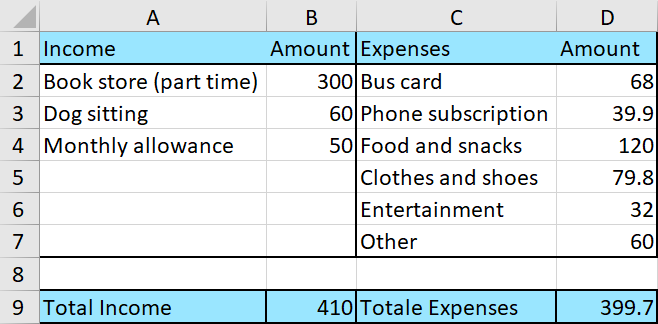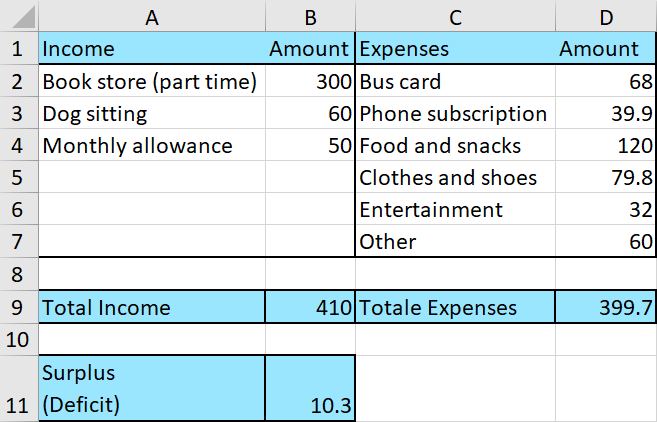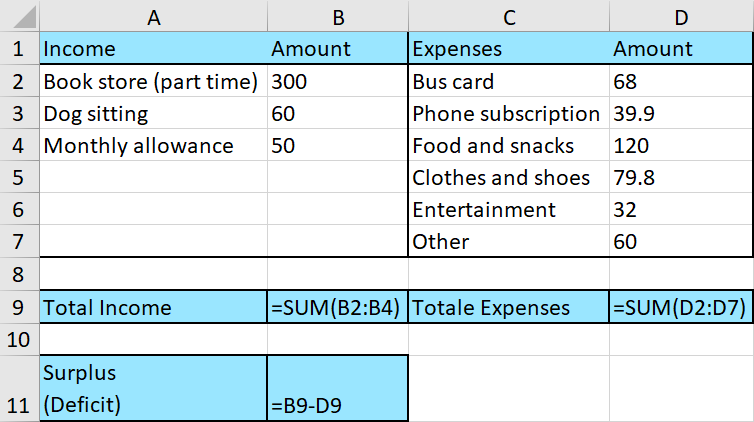# How to Create an Accounting Spreadsheet in Excel

An accounting tells us about our actual income and expenses, in contrast to a Budget, which is a projection of those amounts.

Here is how you proceed when you are making a spreadsheet in `Excel`:

`Excel` Instruction

1.
Divide your various income and expenses into separate categories. These categories will depend on your sources of income (or the company’s). Often, you have already made a budget. Use the same categories as you used in the budget, plus any that were unexpected and you didn’t budget for.
2.
Input the amounts for each category.
3.
4.
Lastly, calculate the actual surplus or deficit by subtracting expenses from income.

Example 1

1.
Erica Clementine has tallied up all of her income and expenses last month. They are as follows:

 Income Amount Expenses Amount Bookstore (part time) \$$300$ Bus card \$$68$ Dog sitting \$$60$ Phone subscription \$$39.90$ Monthly allowance \$$50$ Food and snacks \$$120$ Clothes and shoes \$$79.80$ Entertainment \$$32$ Other \$$60$

2.
Your job is to enter these numbers in `Excel`. Your spreadsheet should look like this:3.
Add up the income, then add up the expenses, to see if Erica Clementine had a surplus or a deficit this month.Erica had a surplus last month of \$$10.30.$

Here are the formulas for each cell: